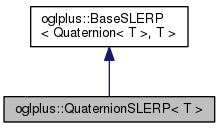OGLplus (0.52.0) a C++ wrapper for OpenGLoglplus::QuaternionSLERP< T > Class Template Reference

Functor template for quaternion spherical-linear interpolation. More...

`#include </home/chochlik/devel/oglplus/include/oglplus/math/quaternion.hpp>`

Inheritance diagram for oglplus::QuaternionSLERP< T >:[legend]
Collaboration diagram for oglplus::QuaternionSLERP< T >:[legend]

## Public Member Functions

QuaternionSLERP (const Quaternion< T > &q1, const Quaternion< T > &q2, T eps=0.001)
Constructs a SLERP functor from two unit quaternions. More...

Quaternion< Toperator() (T param) const
Interpolates between the values passed to constructor. More...

## Detailed Description

### template<typename T> class oglplus::QuaternionSLERP< T >

Functor template for quaternion spherical-linear interpolation.

QuaternionSLERP can be used to smoothly interpolate between two unit quaternions.

## Constructor & Destructor Documentation

template<typename T >
 oglplus::QuaternionSLERP< T >::QuaternionSLERP ( const Quaternion< T > & q1, const Quaternion< T > & q2, T eps = `0.001` )

Constructs a SLERP functor from two unit quaternions.

Precondition
q1.IsNormal() && q2.IsNormal()

## Member Function Documentation

 Quaternion< T > oglplus::BaseSLERP< Quaternion< T > , T >::operator() ( T param ) const
inherited

Interpolates between the values passed to constructor.

Precondition
(param >= 0) && (param <= 1)

The documentation for this class was generated from the following file: